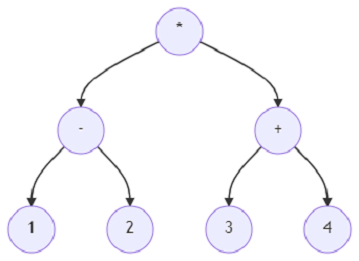# Program to build and evaluate an expression tree using Python

Suppose, we are given the post order traversal of an expression tree. We have to build an expression tree from the given post-order traversal, and then evaluate the expression. We return the root of the expression tree and the evaluated value of the tree.

So, if the input is likethen the output will be -7.

The postfix order given as input of the tree is ['1', '2', '+', '3', '4', '+', '*']. The expression if evaluated, becomes (1 – 2) * (3 + 4); which equals -7.

To solve this, we will follow these steps −

• LEFT = 0 RIGHT = 1

• Define a function evaluate() . This will take root

• if value of root is a numeric value, then

• return integer representation of value of root

• left_val := evaluate(left of root)

• right_val := evaluate(right of root)

• if value of root = '+', then

• return left_val + right_val

• otherwise when value of root = '-', then

• return left_val - right_val

• otherwise when value of root = '*', then

• return left_val * right_val

• otherwise when value of root = '/', then

• return floor value of (left_val / right_val)

• Define a function buildTree() . This will take postfix

• root := null

• stack := a new list

• while postfix is not empty, do

• curr := delete last element from postfix

• curr_node := a new node containing the value curr

• if root is empty, then

• root := curr_node

• if stack is not empty, then

• parent := delete last element from stack

• side := parent

• if side is same as LEFT, then

• left of parent := curr_node

• otherwise,

• right of parent := curr_node

• if curr is not a numerical value, then

• insert tuple (curr_node, LEFT) at the end of stack

• insert tuple(curr_node, RIGHT) at the end of stack

• return root

Let us see the following implementation to get better understanding −

## Example

LEFT = 0
RIGHT = 1
class Node():
def __init__(root, val, left = None, right = None):
root.val = val
root.left = left
root.right = right
def evaluate(root):
if(root.val.isnumeric()):
return int(root.val)
left_val = evaluate(root.left)
right_val = evaluate(root.right)
return (
( root.val == '+' and left_val + right_val ) or
( root.val == '-' and left_val - right_val ) or
( root.val == '*' and left_val * right_val ) or
( root.val == '/' and left_val // right_val )
)
def buildTree(postfix):
root = None
stack = []
while(postfix):
curr = postfix.pop()
curr_node = Node(curr)
if(not root):
root = curr_node
if(stack):
parent, side = stack.pop()
if(side == LEFT):
parent.left = curr_node
else:
parent.right = curr_node
if(not curr.isnumeric()):
stack.append((curr_node, LEFT))
stack.append((curr_node, RIGHT))
return root
root = buildTree(['1', '2', '+', '3', '4', '+', '*'])
print(evaluate(root))

## Input

['1', '2', '+', '3', '4', '+', '*']

## Output

-7